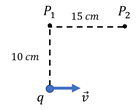# Problem: A 15 C charge moves at a speed of 150 m/s in the –x-direction. What would be the magnitude and direction of the magnetic field at position P1 in the following figure? What about at position P2 in the figure?

###### FREE Expert Solution
88% (153 ratings)
###### Problem Details

A 15 C charge moves at a speed of 150 m/s in the –x-direction. What would be the magnitude and direction of the magnetic field at position P1 in the following figure? What about at position P2 in the figure?